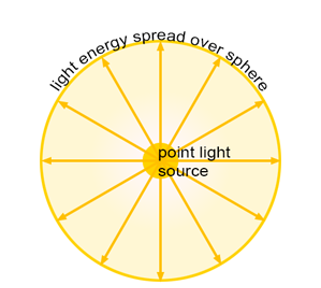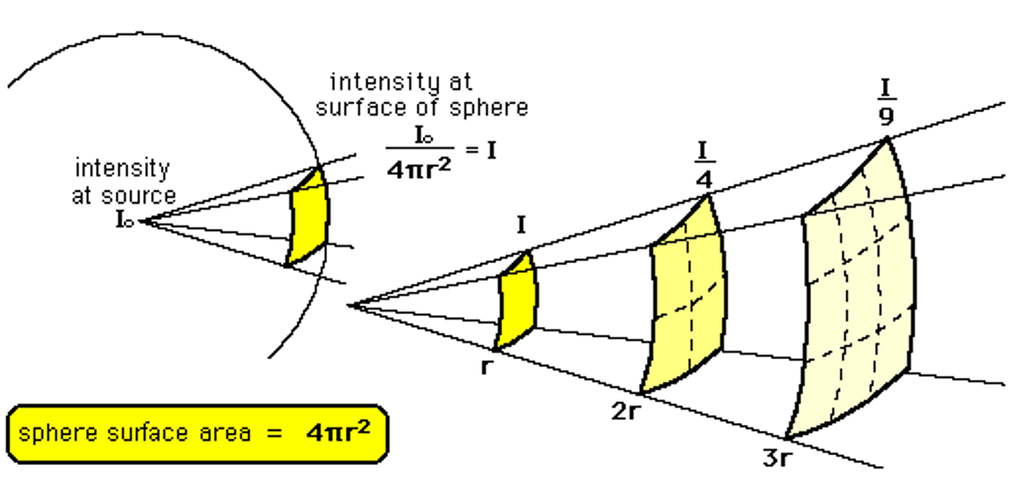# Intensity of Light & Inverse Square Law

This topic is part of the HSC Physics course under the section Ray Model of Light.

### HSC Physics Syllabus

• conduct an investigation to demonstrate the relationship between inverse square law, the intensity of light and the transfer of energy (ACSPH077)

### Understanding Light Intensity

In basic terms, the intensity of light at a point refers to the power of the light (in watts) arriving per unit area (typically in square meters) at that point. It quantifies how much energy from the light source strikes the surface each second.

Mathematically, intensity I is defined as:

$$I = \frac{P}{A}$$

Where:

• I is the intensity in watts per square meter (W/m2).
• P is the power of the light in watts (W).
• A is the area over which the power is distributed in square meters (m2).

### The Inverse Square Law

The inverse square law is a principle that states that the intensity of light radiating from a point source diminishes with the square of the distance from the source. This means, if you double the distance from the light source, the intensity becomes a quarter of its initial value.For a light source that radiates outward, the area it covers can be modelled as a sphere. Therefore, the equation can be modified by using the surface area of a sphere (A = 4 \pi r^2):

$$I = \frac{P}{4\pi r^2}$$

Where:

• r is the distance from the light source.

The following diagram will help you visualise the equation above. As the distance from the source of light increases, the light energy is spread over an increasing area, resulting in a decreasing intensity. Inverse square law for intensity not only applies to light but other electromagnetic waves such as radio waves.By rearranging the equation, the power of light is given by:

$$P = I \times 4\pi r^2$$

If the power of the light source remains constant, that is if the rate of energy transfer remains unchanged, the following expression is true:

$$I_1 \times 4\pi {r_1}^2 = I_2 \times 4\pi {r_2}^2$$

$$I_1 {r_1}^2 = I_2 {r_2}^2$$

### Energy Transfer and Light Intensity

Light carries energy. When it falls upon a surface, this energy is transferred to that surface. The greater the intensity of light hitting the surface, the more energy is transferred to that surface in a given time.

To understand the direct relationship between intensity and energy transfer, consider the equation:

$$E = I \times A \times t$$

Where:

• E is the energy transferred.
• t is the time for which the light falls on the surface.

### Example 1

A light bulb produces 100 J of energy every 5 seconds. What is the intensity of light measured at 6 m from the light bulb?

Solution:

To calculate the power (P):

$$P = \frac{E}{t}$$

$$P = \frac{100 \, \text{J}}{5 \, \text{s}} = 20 \, \text{W}$$

Using the inverse square law to find the intensity (I):

$$I = \frac{P}{4\pi r^2}$$

$$I = \frac{20}{4\pi (6^2)} = 0.0442 \, W \, m^{-2}$$

### Example 2

The intensity of a radio signal is 0.120 W m–2 at 16 m from a small transmitter. What is the intensity of the signal 4.0 m from the transmitter?

Solution:

$$I_1 {r_1}^2 = I_2 {r_2}^2$$

$$(0.120)(16^2)= I_2 (4)^2$$

$$I_2 = 1.92 \, W \, m^{-2}$$